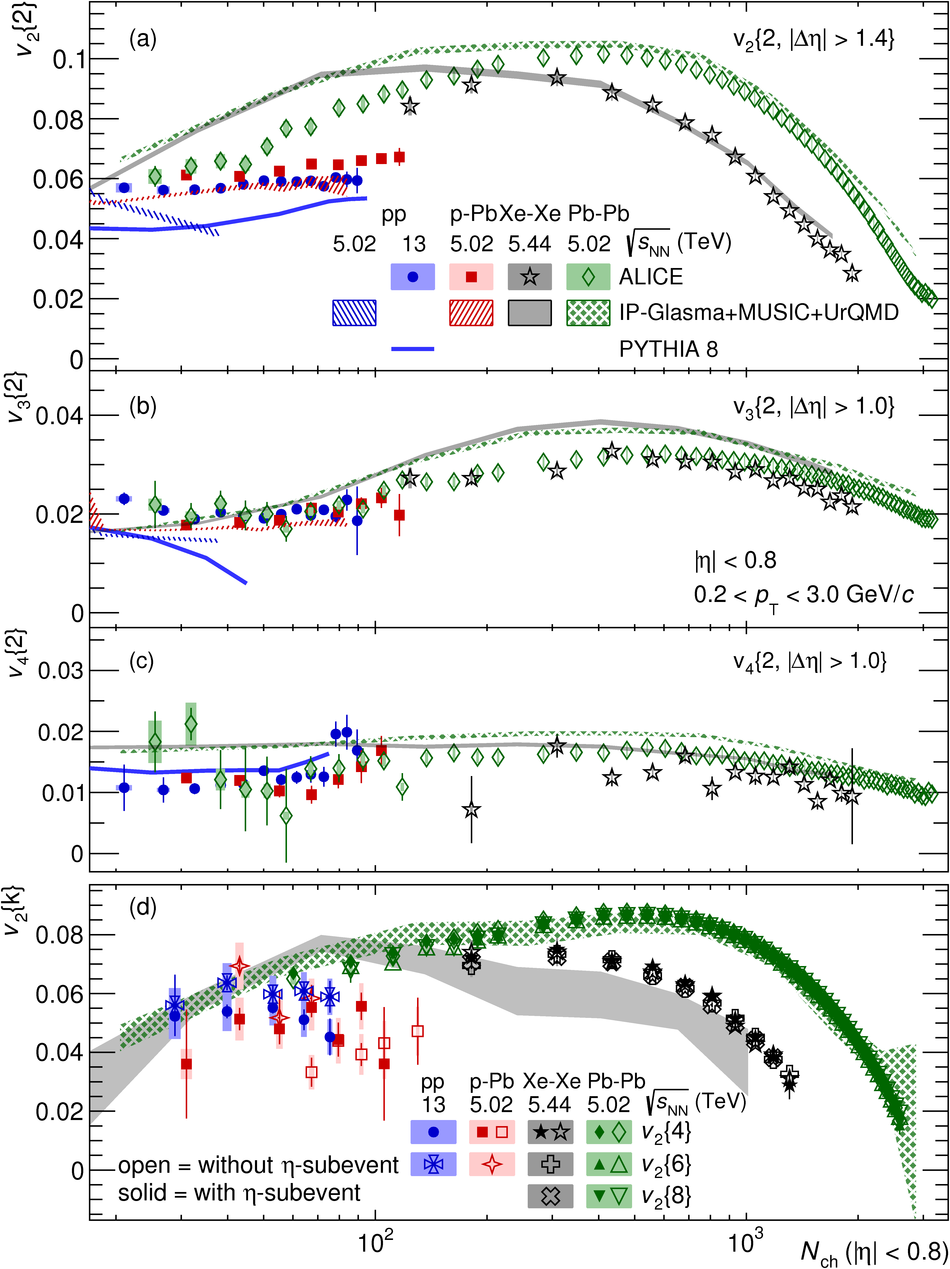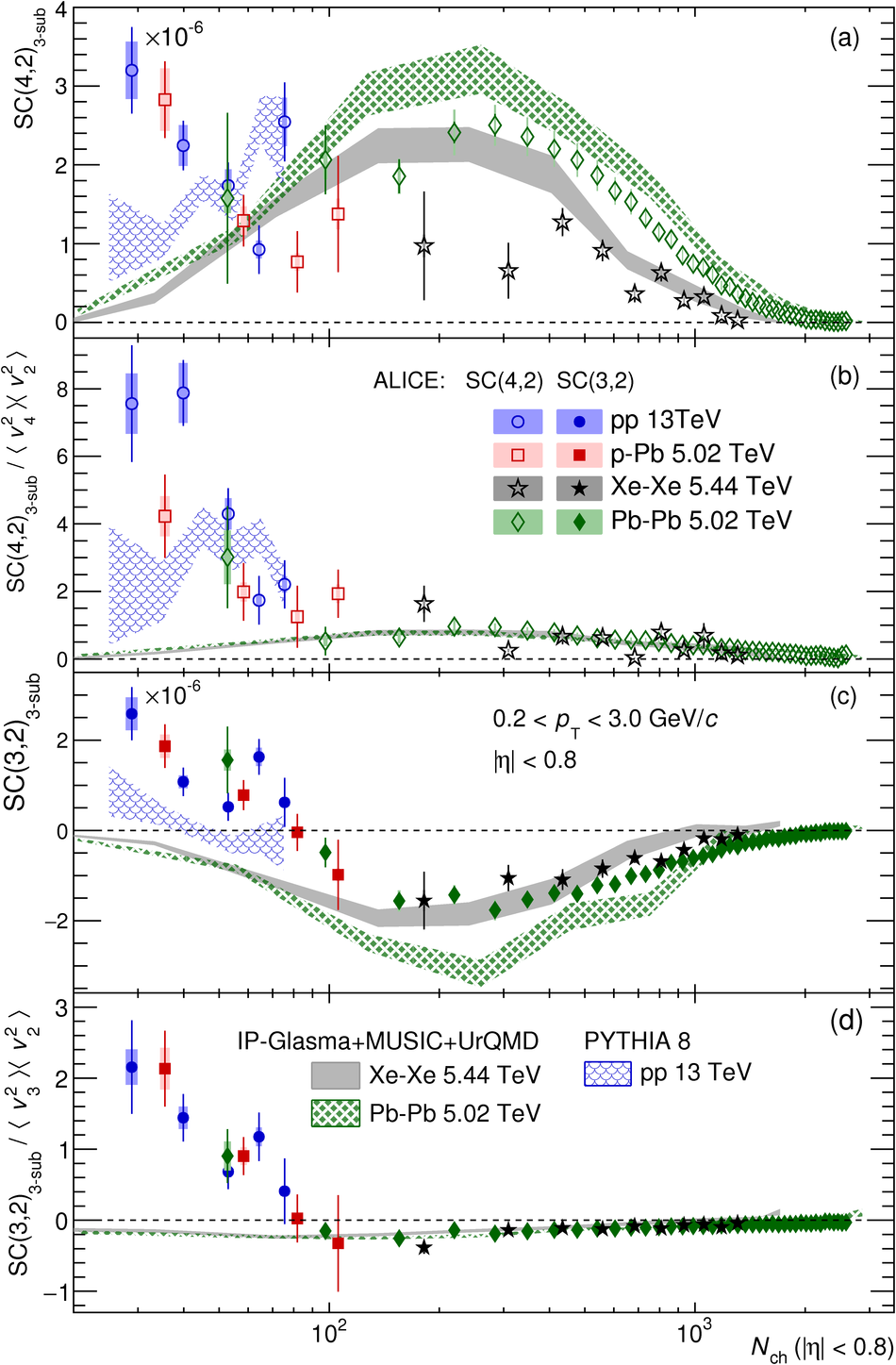# Investigations of anisotropic flow using multi-particle azimuthal correlations in pp, p-Pb, Xe-Xe, and Pb-Pb collisions at the LHC

Measurements of anisotropic flow coefficients ($v_n$) and their cross-correlations using two- and multi-particle cumulant methods are reported in collisions of pp at $\sqrt{s} = 13$ TeV, p-Pb at $\sqrt{s_{_{\rm NN}}} = 5.02$ TeV, Xe-Xe at $\sqrt{s_{_{\rm NN}}} = 5.44$ TeV, and Pb-Pb at $\sqrt{s_{_{\rm NN}}} = 5.02$ TeV recorded with the ALICE detector. The multiplicity dependence of $v_n$ is studied in a very wide range from 20 to 3000 particles produced in the mid-rapidity region $|\eta|<~0.8$ for the transverse momentum range $0.2 <~ p_{\rm T} <~ 3.0$ GeV/$c$. An ordering of the coefficients $v_2 > v_3 > v_4$ is found in pp and p-Pb collisions, similar to that seen in large collision systems, while a weak $v_2$ multiplicity dependence is observed relative to nucleus-nucleus collisions in the same multiplicity range. Using a novel subevent method, $v_{2}$ measured with four-particle cumulants is found to be compatible with that from six-particle cumulants in pp and p-Pb collisions. The magnitude of the correlation between $v_n^2$ and $v_m^2$, evaluated with the symmetric cumulants SC$(m,n)$ is observed to be positive at all multiplicities for $v_2$ and $v_4$, while for $v_2$ and $v_3$ it is negative and changes sign for multiplicities below 100, which may indicate a different $v_n$ fluctuation pattern in this multiplicity range. The observed long-range multi-particle azimuthal correlations in high multiplicity pp and p-Pb collisions can neither be described by PYTHIA 8 nor by IP-Glasma+MUSIC+UrQMD model calculations, and hence provide new insights into the understanding of collective effects in small collision systems.

Figures

## Figure 1

 (coloured online) Multiplicity dependence of $v_n\{k\}$ for \pp, \pPb, \XeXe\ and \PbPb\ collisions. Statistical uncertainties are shown as vertical lines and systematic uncertainties as filled boxes Data are compared with PYTHIA 8.210 Monash 2013~ simulations (solid lines) of pp collisions at $\sqrt{s} = 13$ TeV and IP-Glasma+MUSIC+UrQMD~ calculations of pp, \pPb, \PbPb\ collisions at $\snn = 5.02$ TeV and \XeXe\ collisions at $\snn = 5.44$ TeV (filled bands). The width of the band represents the statistical uncertainty of the model (a), (b) and (c): $v_2$, $v_3$ and $v_4$ measured using two-particle cumulants with a pseudorapidity separation $|\Delta\eta| > 1.4$, 1.0 and 1.0, respectively. (d): $v_2$ measured using multi-particle cumulants, with the 3-subevent method for the four-particle cumulant, and 2-subevent method for higher order cumulants in \PbPb\ collisions.## Figure 2

 (coloured online) Multiplicity dependence of the (a) and (c) symmetric cumulant $SC(m,n)_{\rm{3-sub}}$ and (b) and (d) normalized ratio SC$(m,n)_{\rm{3-sub}} / \langle v_m^2\rangle\langle v_n^2\rangle$ for \pp, \pPb, \XeXe\ and \PbPb\ collisions. Observables in the denominator are obtained from the $v_2\{2, |\Delta\eta| > 1.4\}$ and $v_n\{2, |\Delta\eta| > 1.0\}$ for higher harmonics. Statistical uncertainties are shown as vertical lines and systematic uncertainties as filled boxes. The measurements in large collision systems are compared with the IP-Glasma+MUSIC+UrQMD~ calculations and results in pp collisions are compared with the PYTHIA 8 model~.